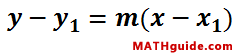The Point-Slope Formula
View the Lesson | MATHguide homepage Updated December 18th, 2016

Status: Waiting for your answers.Use the point and slope formula above to solve this problem.

 Given: The slope of a line is -1/5 and the point on the same line is (-20,0). Find: The equation of the line in slope-intercept form, y = mx + b.     y = -3/2 -3/5 -3/10 -1/2 -1/5 -1/10 1/2 1/5 1/10 3/2 3/5 3/10 x +